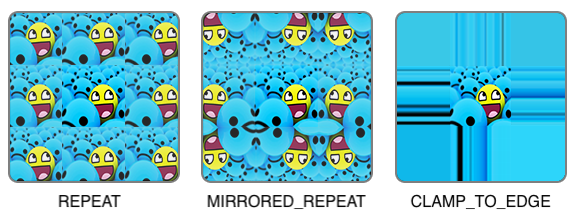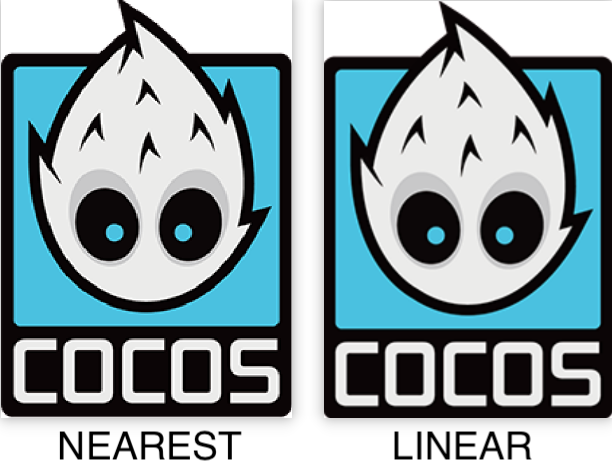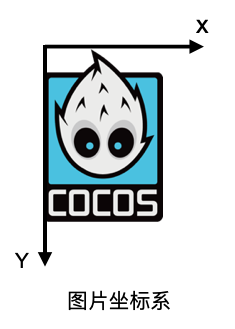``````const uvs = [
0, 0, // 左下角
0, 1, // 左上角
1, 0, // 右下角
1, 1 // 右上角
];
``````

``````// 可以通过 gl.texParameter[fi] 对坐标不同轴向设置（2D 纹理 st 对应 uv，3D 纹理 str 对应 uvw ）
// void gl.texParameterf(target, pname, param);
// 参数请参考：https://developer.mozilla.org/zh-CN/docs/Web/API/WebGLRenderingContext/texParameter
// 由于应用条件较多，可以直接上链接了解一下，然后对应理解教程里涉及的部分即可
gl.texParameteri(gl.TEXTURE_2D, gl.TEXTURE_WRAP_S, gl.CLAMP_TO_EDGE);
gl.texParameteri(gl.TEXTURE_2D, gl.TEXTURE_WRAP_T, gl.CLAMP_TO_EDGE);
``````• NEAREST 临近过滤 （下图左）：选择中心点最接近纹理坐标的那个像素，也是最简单的纹理过滤方式，效率最高。

• LINEAR 线性过滤 （下图右）：选择中心点周围最近的 4 个纹素加权计算出来，一个纹理像素的中心距离纹理坐标越近，那么这个纹理像素的颜色对最终的样本颜色的贡献越大。``````// 当进行缩小时
gl.texParameteri(gl.TEXTURE_2D, gl.TEXTURE_MIN_FILTER, gl.NEAREST);
// 当进行放大时
gl.texParameteri(gl.TEXTURE_2D, gl.TEXTURE_MAG_FILTER, gl.LINEAR);
``````

``````function createShader(gl, type, source) {
// ...
}

// ...
}

function main() {
const image = new Image();
// 如果是用 WebGL 中文文档上内置的运行环境编辑内容的，可以直接用网站内置的纹理图片。https://webglfundamentals.org/webgl/resources/leaves.jpg。
// 由于我这里是自定义了本地的文件，因此创建了一个本地服务器来加载图片。使用本地文件的方式在文章末尾处。
image.src = "http://192.168.55.63:8080/logo.png";
render(image);
};
}

function render() {
const canvas = document.createElement('canvas');
document.getElementsByTagName('body').appendChild(canvas);
canvas.width = 400;
canvas.height = 300;

const gl = canvas.getContext("webgl");
if (!gl) {
return;
}

attribute vec2 a_position;
// 纹理贴图 uv 坐标
attribute vec2 a_uv;
attribute vec4 a_color;
varying vec4 v_color;
varying vec2 v_uv;
// 着色器入口函数
void main() {
v_color = a_color;
v_uv = a_uv;
gl_Position = vec4(a_position, 0.0, 1.0);
}`;

// 让顶点的比例和图像比例一致
const ratio = (image.width / image.height) / (canvas.width / canvas.height);
const positions = [
-ratio, -1,
-ratio, 1,
ratio, -1,
ratio, 1
];

const uvs = [
0, 0, // 左下角
0, 1, // 左上角
1, 0, // 右下角
1, 1 // 右上角
];

// 在片元着色器文本处暂时屏蔽颜色带来的影响，但此处颜色值我们还是上传给顶点着色器
const colors = [
255, 0, 0, 255,
0, 255, 0, 255,
0, 0, 255, 255,
255, 127, 0, 255
];

const indices = [
0, 1, 2,
2, 1, 3
];

const vertexBuffer = gl.createBuffer();
gl.bindBuffer(gl.ARRAY_BUFFER, vertexBuffer);

const attribOffset = (positions.length + uvs.length) * Float32Array.BYTES_PER_ELEMENT + colors.length;
const arrayBuffer = new ArrayBuffer(attribOffset);
const float32Buffer = new Float32Array(arrayBuffer);
const colorBuffer = new Uint8Array(arrayBuffer);
// 当前顶点属性结构方式是 pos + uv + color
// 按 float 32 分布 pos（2）+ uv（2） + color（1）
// 按子节分布 pos（2x4） + uv（2x4） + color（4）
let offset = 0;
let i = 0;
for (i = 0; i < positions.length; i += 2) {
float32Buffer[offset] = positions[i];
float32Buffer[offset + 1] = positions[i + 1];
offset += 5;
}

offset = 2;
for (i = 0; i < uvs.length; i += 2) {
float32Buffer[offset] = uvs[i];
float32Buffer[offset + 1] = uvs[i + 1];
offset += 5;
}

offset = 16;
for (let j = 0; j < colors.length; j += 4) {
// 2 个 position 的 float，加 4 个 unit8，2x4 + 4 = 12
// stride + offset
colorBuffer[offset] = colors[j];
colorBuffer[offset + 1] = colors[j + 1];
colorBuffer[offset + 2] = colors[j + 2];
colorBuffer[offset + 3] = colors[j + 3];
offset += 20;
}

gl.bufferData(gl.ARRAY_BUFFER, arrayBuffer, gl.STATIC_DRAW);

const indexBuffer = gl.createBuffer();
gl.bindBuffer(gl.ELEMENT_ARRAY_BUFFER, indexBuffer);
gl.bufferData(gl.ELEMENT_ARRAY_BUFFER, new Uint16Array(indices), gl.STATIC_DRAW);
precision mediump float;
varying vec2 v_uv;
varying vec4 v_color;
// GLSL 有一个供纹理对象使用的内建数据类型，叫做采样器(Sampler)，它以纹理类型作为后缀
// 比如此处使用的是 2D 纹理，类型就定义为 sampler2D
uniform sampler2D u_image;
// 着色器入口函数
void main() {
// 使用 GLSL 内建函数 texture2D 采样纹理，它第一个参数是纹理采样器，第二个参数是对应的纹理坐标
// 函数会使用之前设置的纹理参数对相应的颜色值进行采样，这个片段着色器的输出就是纹理的（插值）纹理坐标上的（过滤后的）颜色。
gl_FragColor = texture2D(u_image, v_uv);
}`;
gl.viewport(0, 0, gl.canvas.width, gl.canvas.height);
gl.clearColor(0, 0, 0, 255);
gl.clear(gl.COLOR_BUFFER_BIT);
gl.useProgram(program);
const positionAttributeLocation = gl.getAttribLocation(program, "a_position");
gl.enableVertexAttribArray(positionAttributeLocation);
const uvAttributeLocation = gl.getAttribLocation(program, "a_uv");
gl.enableVertexAttribArray(uvAttributeLocation);
const colorAttributeLocation = gl.getAttribLocation(program, "a_color");
gl.enableVertexAttribArray(colorAttributeLocation);
gl.bindBuffer(gl.ARRAY_BUFFER, vertexBuffer);
gl.vertexAttribPointer(positionAttributeLocation, 2, gl.FLOAT, false, 20, 0);
// 新增顶点属性纹理坐标，这里大家应该都很清楚了，就不再多说了
gl.vertexAttribPointer(uvAttributeLocation, 2, gl.FLOAT, false, 20, 8);
gl.vertexAttribPointer(colorAttributeLocation, 4, gl.UNSIGNED_BYTE, true, 20, 16);
const texture = gl.createTexture();
gl.bindTexture(gl.TEXTURE_2D, texture);
// 设置纹理的环绕方式
gl.texParameteri(gl.TEXTURE_2D, gl.TEXTURE_WRAP_S, gl.CLAMP_TO_EDGE);
gl.texParameteri(gl.TEXTURE_2D, gl.TEXTURE_WRAP_T, gl.CLAMP_TO_EDGE);
// 设置纹理的过滤方式
gl.texParameteri(gl.TEXTURE_2D, gl.TEXTURE_MIN_FILTER, gl.NEAREST);
gl.texParameteri(gl.TEXTURE_2D, gl.TEXTURE_MAG_FILTER, gl.LINEAR);
// gl.texImage2D(target, level, internalformat, format, type, HTMLImageElement? pixels);
// 此接口主要为了指定二维纹理图像，图像的来源有多种，可以直接采用 HTMLCanvasElement、HTMLImageElement 或者 base64。此处选用最基础的 HTMLImageElement 讲解。
// 关于参数的详细内容请参考：https://developer.mozilla.org/zh-CN/docs/Web/API/WebGLRenderingContext/texImage2D
gl.texImage2D(gl.TEXTURE_2D, 0, gl.RGBA, gl.RGBA, gl.UNSIGNED_BYTE, image);
gl.bindBuffer(gl.ELEMENT_ARRAY_BUFFER, indexBuffer);
gl.drawElements(gl.TRIANGLES, indices.length, gl.UNSIGNED_SHORT, 0);
}
``````

``````// 翻转图片
gl.pixelStorei(gl.UNPACK_FLIP_Y_WEBGL, true);
````````````function main() {
// 新增加一张纹理贴图
const images = ["http://192.168.55.63:8080/logo.png", "http://192.168.55.63:8080/close-icon.png"];
const dataList = [];
let index = 0;
for (let i = 0; i < 2; i++) {
const image = new Image();
image.src = images[i];
dataList.push(image);
index++;
if (index >= images.length) {
render(dataList);
}
};
}
}

function render(dataList) {
// ...
// 重新定义顶点位置
const ratio = 0.5;
const positions = [
-ratio, -1,
-ratio, 1,
ratio, -1,
ratio, 1
];
// ...

// 修改片元着色器文本
precision mediump float;
varying vec2 v_uv;
varying vec4 v_color;
// 新增一个纹理
uniform sampler2D u_image0;
uniform sampler2D u_image1;
// 着色器入口函数
void main() {
vec4 tex1 = texture2D(u_image0, v_uv);
vec4 tex2 = texture2D(u_image1, v_uv);
// 将纹理色值相乘
// rgb 和黑色相乘都为黑色（黑色 rgb 每分量都是 0），和白色相乘，都为原色（白色 rbg 每分量都是 1）
gl_FragColor = tex1 * tex2;
}`;

// ...

// 判断有纹理才设置翻转
if(dataList.length > 0){
gl.pixelStorei(gl.UNPACK_FLIP_Y_WEBGL, true);
}

for (let j = 0; j < dataList.length; j++) {
const data = dataList[j];
const samplerName = `u_image\${j}`;
const u_image = gl.getUniformLocation(program, samplerName);
// 设置每个纹理的位置值
gl.uniform1i(u_image, j);
const texture = gl.createTexture();
gl.activeTexture(gl.TEXTURE0 + j);
gl.bindTexture(gl.TEXTURE_2D, texture);
gl.texParameteri(gl.TEXTURE_2D, gl.TEXTURE_WRAP_S, gl.CLAMP_TO_EDGE);
gl.texParameteri(gl.TEXTURE_2D, gl.TEXTURE_WRAP_T, gl.CLAMP_TO_EDGE);
gl.texParameteri(gl.TEXTURE_2D, gl.TEXTURE_MIN_FILTER, gl.NEAREST);
gl.texParameteri(gl.TEXTURE_2D, gl.TEXTURE_MAG_FILTER, gl.LINEAR);
gl.texImage2D(gl.TEXTURE_2D, 0, gl.RGBA, gl.RGBA, gl.UNSIGNED_BYTE, data);
}
}
``````

``````// 此处展示出部分应用代码

const colors = [
255, 0, 0, 255,
0, 255, 0, 255,
0, 0, 255, 255,
255, 127, 0, 255
];

precision mediump float;
varying vec2 v_uv;
varying vec4 v_color;
uniform sampler2D u_image;
void main() {
vec4 tex1 = texture2D(u_image, v_uv);
gl_FragColor = tex1 * v_color;
}`;
````````````const fragmentShaderSource = `
precision mediump float;
varying vec2 v_uv;
varying vec4 v_color;
uniform sampler2D u_image;
void main() {
vec4 tex1 = texture2D(u_image, v_uv).bgra;
gl_FragColor = tex1;
}`;
````````````npm install http-server -g

// 安装完成之后执行
http-server
// 此时控制台会出现类似如下：
Available on:
http://127.0.0.1:8080
http://192.168.55.63:8080
``````

# 要看下一集？戳这里看全系列教程>>>>

4赞

vec3 blendHSL = RGBToHSL(vec3(blendColor.r,blendColor.g,blendColor.b));

// varying vec4 v_fragmentColor;
// vec4 target_color = v_fragmentColor * vec4(baseColor.r , baseColor.g , baseColor.b , textureColor.a);

blendColor[cc.2.4.0].zip (4.4 KB)